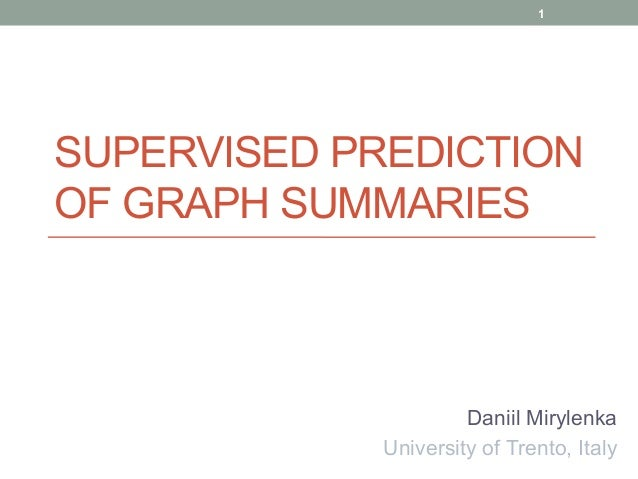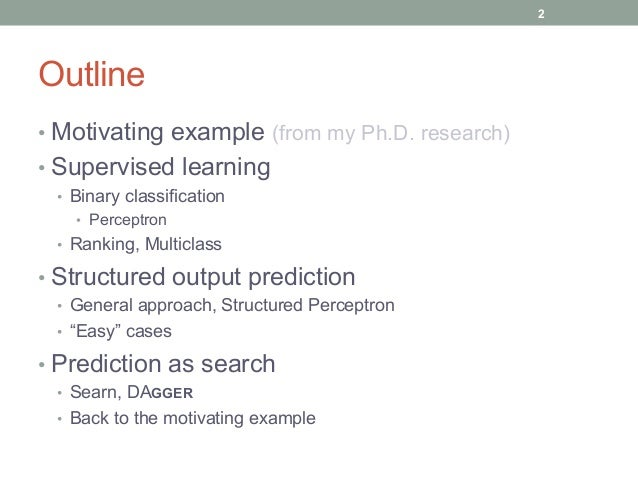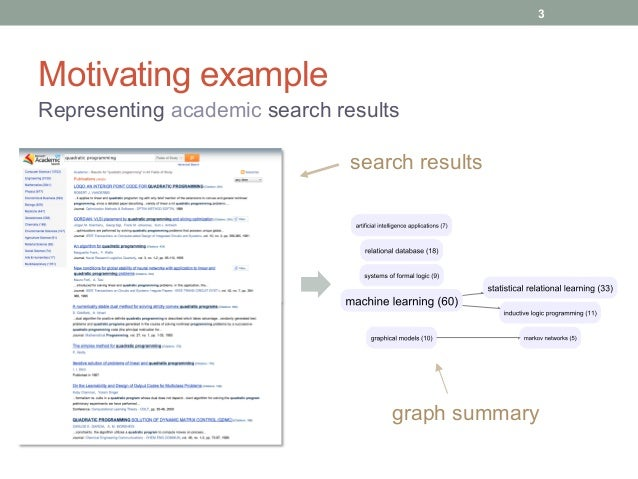Successfully reported this slideshow.Upcoming SlideShare
×

ofUpcoming SlideShare
Nlp.set
Next

0 Likes

Share

# Supervised Prediction of Graph Summaries

Supervised machine learning addresses the problem of approximating a function, given the examples of inputs and outputs. The classical tasks of regression and classification deal with functions whose outputs are real numbers. Structured output prediction goes beyond one-dimensional outputs, and allows predicting complex objects, such as sequences, trees, and graphs. In this talk I will show how to apply structured output prediction to building informative summaries of the topic graphs—a problem I encountered in my Ph.D. research. The focus of the talk will be on understanding the intuitions behind the machine learning algorithms. We will start from the basics and walk our way through the inner workings of DAgger—state-of-the-art method of structured output prediction.

This talk was be given at a seminar in Google Krakow.

See all

### Related Audiobooks

#### Free with a 30 day trial from Scribd

See all
• Be the first to like this

### Supervised Prediction of Graph Summaries

1. 1. SUPERVISED PREDICTION OF GRAPH SUMMARIES Daniil Mirylenka University of Trento, Italy 1
2. 2. Outline • Motivating example (from my Ph.D. research) • Supervised learning •  Binary classification •  Perceptron •  Ranking, Multiclass • Structured output prediction •  General approach, Structured Perceptron •  “Easy” cases • Prediction as search •  Searn, DAGGER •  Back to the motivating example 2
3. 3. Motivating example Representing academic search results 3 graph summary search results
4. 4. Motivating example Suppose we can do this: 4 large graph
5. 5. Motivating example Then we only need to do this: 5 small graph
6. 6. Motivating example What is a good graph summary? Let’s learn from examples! 6
7. 7. Supervised learning 7
8. 8. What is supervised learning? x1, y1( ),  x2, y2( ), …,  xn, yn( ) f : x ! y 8 Supervised Learning bunch of examples function
9. 9. x1, y1( ),  x2, y2( ), …,  xn, yn( ) 9 distribution of examples Statistical learning theory P(x,y) Where do our examples come from? samples drawn i.i.d.
10. 10. 10 hypothesis space Statistical learning theory What functions do we consider? f ∈ H linear? H1 cubic? H2 piecewise-linear? H3
11. 11. 11 loss function Statistical learning theory How bad is it to predict instead of (true) ?f x( ) y L f x( ), y( ) L y, !y( )= 0, when y = !y 1, otherwise " # \$ Example: zero-one loss
12. 12. Statistical learning theory argmin f ∈H L( f (x), y)p(x, y)dxdy X×Y ∫ Goal: Requirement: argmin f ∈H L( f (xi ), yi ) i=1 n ∑ 12 expected loss on new examples total loss on training data
13. 13. Linear models w = argmin w L( fw x( ), yi ) i=1 n ∑ Inference (prediction): fw x( )= g( w,ϕ x( ) ) optimization with respect to w (e.g. gradient descent) Learning: 13 features of x scalar product (linear combination, weighted sum) H
14. 14. Binary classification y ∈ {−1,1} Prediction: fw x( )= sign( w, x ) 14 above or below the line (hyperplane)? yi w, xi > 0 Note that: w, x > 0 w, x < 0 w, x = 0
15. 15. Perceptron Learning algorithm (optimizes one example at a time) For every xi if yi w, xi ≤ 0 w ← w + yi xi •  if made a mistake •  update the weights if yi>0 makes wi+1 more like xi if yi<0 makes wi+1 more like -xi 15 Repeat
16. 16. Perceptron Update rule: wold 16 xi w ← w + yi xi
17. 17. Perceptron wold 17 xi wnew Update rule: w ← w + yi xi
18. 18. Max-margin classification Idea: ensure some distance form the hyperplane 18 yi w, xi ≥1 Require:
19. 19. Preference learning Suppose we want to predict rankings: x ! y = v1 v2 " vk x,vi( )≻ x,vj( )⇔ i < j 19 joint features of x and v w ϕ x,v1( ) ϕ x,v2( ) ϕ x,v3( ) ϕ x,v4( ) ϕ x,v5( ) Also works for: •  selecting just the best one •  multiclass classification w,ϕ x,v( )−ϕ x, "v( ) ≥1
20. 20. Structured prediction 20
21. 21. Structured prediction Examples: •  “Time flies like an arrow.”x = part-of-speech tagging •  (noun verb preposition determiner noun)!y = •  (S (NP (NNP Time)) (VP (VBZ flies) (PP (IN like) (NP (DT an) (NN arrow))))) y = parse tree or 21
22. 22. Structured prediction How can we approach this problem? f x( )= g w,ϕ x( )( )•  before we had: •  now must be a complex objectf x( ) f x( )= argmax y w,ψ x, y( )( ) joint features of x and y (kind of like we had with ranking) 22
23. 23. Structured Perceptron Almost the same as ordinary perceptron •  For every xi •  if ˆyi ≠ yi w ← w +ψ xi, yi( )−ψ xi, ˆyi( ) (if made a mistake) update the weights •  predict: ˆyi = argmax y w,ψ xi, y( )( ) •  23
24. 24. Argmax problem often infeasible ˆy = argmax y w,ψ x, y( )( ) Prediction: Examples: 24 •  sequence of length T, with d options for each label: dT •  a subgraph of size T from a graph G: |G| chose T •  10-word sentence, 5 parts of speech: ~10 million outputs •  10-node subgraph of a 300-node graph: 1,398,320,233,231,701,770 outputs (around 1018)
25. 25. Argmax problem ˆy = argmax y w,ψ x, y( )( ) Prediction: Learning: •  even more difficult •  includes prediction as a subroutine 25 often infeasible
26. 26. Argmax problem: easy cases Independent prediction •  suppose decomposes into •  and decomposes into y v1,v2,…,vT( ) ψ x, y( )= ψi x,vi( ) i=1 T ∑ ψ x, y( ) argmax y w,ψ x, y( ) =               argmax v1 w,ψ1 x,v1( ) ,…,argmax vn w,ψn x,vn( ) ! " # \$ % & •  then predictions can be made independently 26
27. 27. Argmax problem: easy cases Sequence labeling •  suppose decomposes into •  and decomposes into y v1,v2,…,vT( ) ψ x, y( )= ψi x,vi,vi+1( ) i=1 T−1 ∑ ψ x, y( ) •  dynamic programming : O(Td2) 27 •  with ternary features : O(Td3), etc. •  in general tractable in graphs with bounded treewidth
28. 28. Approximate argmax General idea: •  search in the space of outputs Natural generalization: •  space of partial outputs •  composing the solution sequentially Let’s learn to make good moves! How do we decide which moves to take? Most interesting/crazy idea of this talk (And we don’t need the original argmax problem anymore) 28
29. 29. Learning to search 29
30. 30. Learning to search Sequential prediction of structured outputs y = v1,v2,…,vT( )•  decompose the output π : v1,v2,…,vt( )→ vt+1•  learn the policy state action s0 π ! →! s1 π ! →! … π ! →! sT = y •  apply the policy sequentially st → vt+1 •  policy can be trained on examples st,vt+1( ) •  preference learning 30
31. 31. Learning to search The caveat of sequential prediction siStates : coordinates of the car Actions : steering (‘left’, ‘right’)vi+1 Oops! left! left! right! right! left! Problem: •  errors accumulate •  training data is not i.i.d.! Solution: •  Train on the states produced by our policy! Chicken-and-egg problem (solution: iterate) 31
32. 32. Searn and DAGGER Searn = “search” + “learn” •  start from optimal policy; move away •  generate new states with current •  generate actions based on regret •  train on new state-action pairs •  interpolate the current policy πi+1 ← βπi + 1− β( ) #πi+1 policy learnt at ith iteration regret 32  Hal Daumé III, John Langford, Daniel Marcu. Search-based Structured Prediction. Machine Learning Journal, 2006.  !πi+1 πi
33. 33. Searn and DAGGER DAGGER = “dataset” + “aggregation” •  start from the ‘ground truth’ dataset, enrich it with new state-action pairs •  train a policy on the current dataset •  use the policy to generate new states •  generate ‘expert’s actions’ for new states •  add new state-action pairs to the dataset expert’s actions As in Searn, we’ll eventually be training on our own-produced states 33  Stephane Ross, Geoffrey Gordon, Drew Bagnell. A reduction of imitation learning and structured prediction to no-regret online learning. Journal of Machine Learning Research, 2011. 
34. 34. DAGGER for building the graph summaries Input: topic graph , search results , relation Output: topic summary of size G V, E( ) R ⊆ V × S S GT VT , ET( ) T A few tricks: •  Predict only vertices ,VT •  Require that the summaries be nested: •  which means •  hence, the task is to predict the sequence ∅ =V0 ⊂ V1 ⊂…⊂ VT Vi+1 =Vi + vi+1 v1,v2,…,vT( ) 34
35. 35. DAGGER for building the graph summaries •  Provide the ‘ground truth’ topic sequences V,S, R( ),v1,v2,…,vT( ) a single ground truth example topics (vertices) documents (search results) topic-document relations topic sequence •  Create the dataset D0 = ∪ si,vi+1( ){ }i=0 T •  train the policy onπi Di •  apply to the initial states to generate state sequencesπi s0 s1,s2,…,sT( ) empty summary intermediate summaries •  produce the ‘expert action’ for every generated state •  produce v* Di+1 = Di ∪ s,v* ( ){ } 35
36. 36. DAGGER: producing the ‘expert action’ •  The expert’s action brings us closer to the ‘ground-truth’ trajectory expert’s actions •  Suppose the ‘ground-truth’ trajectory is 36 s1,s2,…,sT( ) •  And the generated trajectory is ˆs1, ˆs2,…, ˆsT( ) •  The expert’s action vi+1 * = argmin v Δ ˆsi ∪ v{ },si+1( )( ) dissimilarity between the states
37. 37. DAGGER: topic sequence dissimilarity •  Set-based dissimilarity, e.g. Jaccard distance •  similarity between topics ? •  encourages redundancy •  Sequence-matching based dissimilarity •  greedy approximation 37 Δ v1,v2,…,vt( ), !v1, !v2,…, !vt( )( )
38. 38. DAGGER: topic graph features •  Coverage and diversity •  [transitive] document coverage •  [transitive] topic frequency, average and min •  topic overlap, average and max •  parent-child overlap, average and max •  … 38 ψ V,S, R( ), v1,v2,…,vt( )( )
39. 39. Recap •  We’ve learnt: •  … how to do binary classification •  and implement it in 4 lines of code •  … about more complex problems (ranking, and structured prediction) •  general approach, structured Perceptron •  argmax problem •  … that learning and search are two sides of the same coin •  … how to predict complex structures by building them sequentially •  Searn and DAGGER 39
40. 40. Questions? 40 dmirylenka @ disi.unitn.it
41. 41. Extra slides 41
42. 42. Support Vector Machine Idea: large margin between positive and negative examples Loss function: L y, f x( )( )=[1− y⋅ f x( )]+ Hinge loss (solved by constrained convex optimization) yi w, xi ≥ C C → max # \$ % &% ⇔ yi w, xi ≥1 w → min # \$ % &% 42
43. 43. Structured SVM correct outputs score higher by a margin w → min w,ψ xi, y( ) − w,ψ xi, yi( ) ≥1,  yi ≠ y w,ψ xi, yi( )−ψ xi, y( ) ≥ Δ yi, y( ),  yi ≠ y margin depends on dissimilarity Taking into account (dis)similarity between the outputs 43

Total views

470

On Slideshare

0

From embeds

0

Number of embeds

27

10

Shares

0